# C++ Program to Multiply two Numbers

In this tutorial you will learn about the C++ Program to Multiply two Numbers and its application with practical example.

## C++ Program to Multiply Two Numbers

In this tutorial, we will learn to create a C++ program that will Multiply Two Numbers in C++ programming.

## Prerequisites

Before starting with this tutorial we assume that you are best aware of the following C++ programming topics:

• Operators in C++ Programming.
• Basic Input and Output function in C++ Programming.
• Basic C++ Programming.

## Program to Multiply Two Numbers:-

As we all know the integer, float, or double value in c++ programming. In c++ programming, we can take the input from the user in integer format. In today’s program, we will take input in number format. Secondly, we will Multiply the number with the help of a small program and the arithmetic operator “*”. The numbers are the most useful terminology in any programing language. For arithmetic manipulation in c++ programming, there are many predefined functions available.

Integers are the most use-full data types in any programming language. The integer values are used with operators to perform the best operations in C++ programming.

With the help of this program, we can take input and Multiply Two Numbers.

## Output:-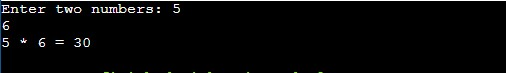In the above program, we have first initialized the required variable.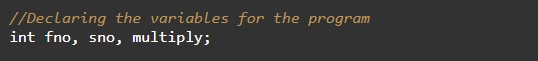• fno = it will hold the input number value from the user.
• sno = it will hold the input number value from the user.
• multiply = it will hold the sum value of the numbers.

Taking Input numbers from the user.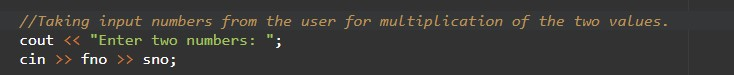Calculating the multiply of two numbers.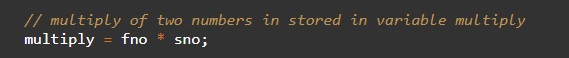Printing the output.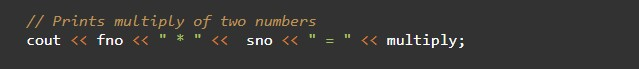In this tutorial we have learn about the C++ Program to Multiply two Numbers and its application with practical example. I hope you will like this tutorial.